# Solving a system of linear equations using Cramer's rule

Cramer's rule may only be applied for a system of linear equations with as many equations as unknowns (the coefficient matrix of the system must be square) and with non-zero determinant of the coefficient matrix.

Consider a system of n linear equations for n unknowns x1, x2, ..., xn:
a11x1 + a12x2 + ...+ a1nxn = b1
a21x1 + a22x2 + ...+ a2nxn = b2
...   ...   ...    ...    ...
an1x1 + an2x2 + ...+ annxn = bn

The determinant of the coefficient matrix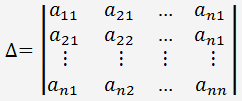Let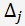be the determinant of the matrix formed by replacing the j column with the column of the constant terms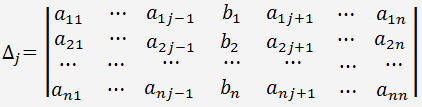If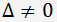, the system has a unique solution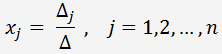Since the computation of large determinants is cumbersome, Cramer's rule is generally used for systems of two and three equations.